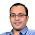### Brownian Motion in Circles Puzzle

Source: http://www.stanford.edu/~gowthamr/puzzles.html

Problem:
Suppose the starting point of a particle undergoing Brownian motion in 2 dimensions is chosen uniformly at random on an imaginary circle C_1. Suppose there is a solid circle C_2 completely inside C_1, not necessarily concentric. Show that the particle hits the boundary of C_2 with uniform distribution.

Book: I strongly recommend the book by Steven Shreve for understanding Brownian Motion and its applications in Financial Modelling (Its expensive! Flipkart Link: Stochastic Calculus for Finance II)

Update (4th Feb 2013):
Solution posted by me in comments!

1.Does the particle 'interact' with any of the circles? How do you count 'hitting' the boundary - from outside, from inside or both?

2.a) The question does not say it interacts, Why would you want to assume any interaction?
b) Since the point is starting from C_1, and C_2 is inside C_1, its impossible to hit C_2 from inside first! So, that doubt also does not require any clarification.

3.This comment has been removed by a blog administrator.

4.Solution posted on Goutham's website: http://www.stanford.edu/~gowthamr/Answer2.html

Assume that the particle starts (at a point randomly chosen with uniform distribution) on the boundary of a circle C_0 of very large radius that is concentric with C_1 . By symmetry, the particle hits C_1 with uniform distribution, hence one may rather assume the particle starts at random from infinity. It is clear then that the particle should hit C_2 with uniform distribution.

5.I'm sorry but I am not able to see the answer on the link posted. Also your answer is not satisfactory. Even if it were coming from infinity I intuitively feel the direction of it would matter a lot. Do you have the mathematical proof for this? In place of BM, if the question talks about any deterministic function, without really talking about the mathematical properties of it, your exact answer could be posted, which would be grossly erroneous.